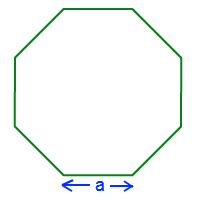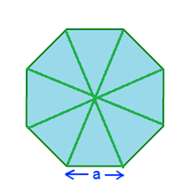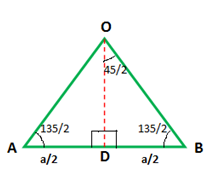# Octagon Formula

A polygon is a two-dimensional (2-D) closed figure made up of straight line segments. In geometry, the octagon is a polygon with 8 sides. If the lengths of all the sides and the measurement of all the angles are equal, the octagon is called a regular octagon. In other words, the sides of a regular octagon are congruent. Each of the interior angle and the exterior angle measure 135° and 45° respectively, in a regular octagon. There is a predefined set of formulas for the calculation of perimeter, and area of a regular octagon which is collectively called as octagon formula. For an octagon with the length of its edge as “a”, the formulas are listed below.Also, check: Octagon

## Octagon Formulas

Formulas for Octagon
Area of an Octagon 2a2(1+√2)
Perimeter of an Octagon 8a

Octagon formula helps us to compute the area and perimeter of octagonal objects.

## Derivation of Octagon Formulas:

Consider a regular octagon with each side “a” units.

### Formula for Area of an Octagon:

Area of an octagon is defined as the region occupied inside the boundary of an octagon.

In order to calculate the area of an octagon, we divide it into small eight isosceles triangles. Calculate the area of one of the triangles and then we can multiply by 8 to find the total area of the polygon.Take one of the triangles and draw a line from the apex to the midpoint of the base to form a right angle. The base of the triangle is a, the side length of the polygon and OD is the height of the triangle.Area of the octagon is given as 8 x Area of Triangle.

2 sin²θ = 1- cos 2θ

2 cos²θ = 1+ cos 2θ

$$\begin{array}{l}tan^{2}\theta = \frac{1-cos2\theta}{1+cos2\theta}\\ tan^{2}(\frac{45}{2})=\frac{1-cos45}{1+cos45}\\ tan^{2}(\frac{45}{2})=\frac{1-\frac{1}{\sqrt{2}}}{1+\frac{1}{\sqrt{2}}}\\ tan^{2}(\frac{45}{2})=\frac{\sqrt{2}-1}{\sqrt{2}+1}=\frac{(\sqrt{2}-1)^{2}}{1}\\ tan(\frac{45}{2})=\sqrt{2}-1\\ \frac{BD}{OD}=\sqrt{2}-1\\ OD=\frac{a/2}{\sqrt{2}-1}=\frac{a}{2}(1+\sqrt{2})\end{array}$$

Area of ∆ AOB =

$$\begin{array}{l}\frac{1}{2}\times AB\times OD\end{array}$$

=

$$\begin{array}{l}\frac{1}{2}\times a\times \frac{a}{2}(1+\sqrt{2})\end{array}$$

=

$$\begin{array}{l}\frac{a^{2}}{4}(1+\sqrt{2})\end{array}$$

Area of the octagon = 8 x Area of Triangle

Area of Octagon =

$$\begin{array}{l}8\times \frac{a^{2}}{4}(1+\sqrt{2})\end{array}$$

Area of an Octagon =

$$\begin{array}{l}2a^{2}(1+\sqrt{2})\end{array}$$

### Formula for Perimeter of an Octagon:

Perimeter of an octagon is defined as the length of the boundary of the octagon. So perimeter will be the sum of the length of all sides. The formula for perimeter of an octagon is given by:

Perimeter = length of 8 sides

So, the perimeter of an Octagon = 8a

### Properties of a Regular Octagon:

• It has eight sides and eight angles.
• Lengths of all the sides and the measurement of all the angles are equal.
• The total number of diagonals in a regular octagon is 20.
• The sum of all interior angles is equal to 1080 degrees, where each interior angle measures 135 degrees.
• The sum of all exterior angles is equal to 360 degrees, where each exterior angle measures 45 degrees.

### Solved examples Using Octagon Formula:

Question 1: Calculate the area and perimeter of a regular octagon whose side is 2.3 cm.

Solution: Given, side of the octagon = 2.3 cm

Area of an Octagon =

$$\begin{array}{l}2a^{2}(1+\sqrt{2})\end{array}$$

Area of an Octagon =

$$\begin{array}{l}2\times 2.3^{2}(1+\sqrt{2})=25.54\;cm^{2}\end{array}$$

Perimeter of the octagon = 8a = 8 × 2.3 = 18.4 cm

Question 2: Perimeter of an octagonal stop signboard is 32 cm. Find the area of the signboard.

Solution: Given,

Perimeter of the stop sign board = 32 cm

Perimeter of an Octagon = 8a

32 cm = 8a

a = 32/8 = 4 cm

Area of an Octagon =

$$\begin{array}{l}2a^{2}(1+\sqrt{2})\end{array}$$

Area of the stop sign board =

$$\begin{array}{l}2\times 4^{2}(1+\sqrt{2})=77.248\;cm^{2}\end{array}$$

To solve more problems on the topic, download BYJU’S – the Learning App.